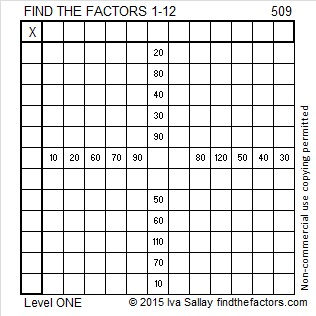# 509 and Level 1

509 = 22^2 + 5^2.

509 is the hypotenuse of the primitive Pythagorean triple, 220-459-509.Print the puzzles or type the solution on this excel file: 12 Factors 2015-06-01

—————————————————————————————————

• 509 is a prime number.
• Prime factorization: 509 is prime.
• The exponent of prime number 509 is 1. Adding 1 to that exponent we get (1 + 1) = 2. Therefore 509 has exactly 2 factors.
• Factors of 509: 1, 509
• Factor pairs: 509 = 1 x 509
• 509 has no square factors that allow its square root to be simplified. √509 ≈ 22.561028How do we know that 509 is a prime number? If 509 were not a prime number, then it would be divisible by at least one prime number less than or equal to √509 ≈ 22.6. Since 509 cannot be divided evenly by 2, 3, 5, 7, 11, 13, 17, or 19, we know that 509 is a prime number.

—————————————————————————————————